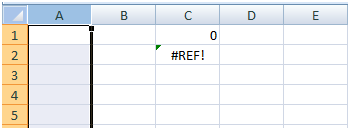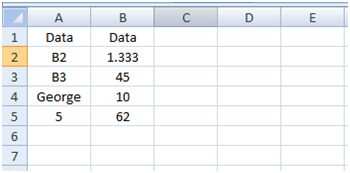# Advance Excel function - Indirect()

@VaibhavJ , 04 September 2014

To understand benefit of indirect function do as I say.

Put 100 in cell A1, now in Cell D1 put formula =INDIRECT("A1") & in cell D2 put formula =A1.Result is same in both case then why to put confusing formula in cell D2?

Now select column A & delete it! What you see now in Cell C1 & C2?So here lies difference, after deleting col A formula in C2 goes off & changes to =#REF! but formula in cell C1 remains intact.

So when you are preparing financial models or projects & you are required to refer to cells & sheets which changes frequently you can use indirect function.

So while moving or copying formula from one workbook to another workbook your reference remains intact in same workbook & not to workbook from the sheet where you moved your data.

Let us see more way of using indirect function.Formula Descriptttttion (Result) =INDIRECT(\$A\$2) Value of the reference in cell A2 (1.333) =INDIRECT(\$A\$3) Value of the reference in cell A3 (45) =INDIRECT(\$A\$4) If the cell B4 has the defined name "George," the value of the defined name is returned (10) =INDIRECT("B"&\$A\$5) Value of a reference in cell A5 (62)

Suppose you have 100 sheets in your workbook name after debtors name & in Cell A1 of each cell there is total amount receivable from each debtors, your boss want to create sheet with Name of Debtors & amount receivable from each Debtors. Task is easy & you can complete it in 30 mins.

But what if there is 500 of such sheets from you need to take values?

With indirect function you can do in 5 mins or in less time, lets see how:

Create header by putting Name in cell A1 & Amount in cell B1.

Now in cola beginning cell A2 put name of sheet, which you can get it from your master data as it is name of your debtors only.

In cell B2 put formula =INDIRECT("'"&A2&"'!A1") & you will get values cell A1 from Sheet named Raj .

Now you just need to drag down / copy paste formula from cell A2 to all cells & you are done.

Do post query / feedback / questions.

Source: Self/Microsoft

@VaibhavJ
Category Info Technology   Report

103 Shares   32211 Views

### Popular ArticlesCCI Articles